## Probability Questions and Answers Part-7

1. Let X and Y be two events such that $P\left( X\mid Y\right)=\frac{1}{2},P\left( Y\mid X\right)=\frac{1}{3}$      and $P\left( X\cap Y\right)=\frac{1}{6}$   Which of the following is(are) correct?
a) $P\left( X\cap Y\right)=\frac{2}{3}$
b) X and Y are independent
c) X and Y are not independent
d) Both a and b

Explanation: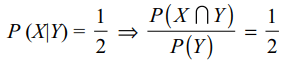2. Three numbers are chosen at random without replacement from {1, 2, ...., 15}. Let $E_{1}$ be the event that minimum of the chosen numbers is 5 and $E_{2}$ their maximum is 10 then
a) $P\left(E_{1}\right)=\frac{9}{91}$
b) $P\left(E_{2}\right)=\frac{36}{455}$
c) $P\left(E_{1} \cap E_{2}\right)=\frac{4}{455}$
d) All of the Above

Explanation: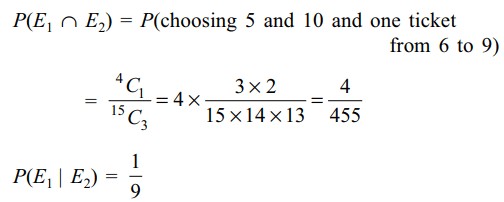3. A group consists 2n students including you and your friend. If the group is split into two different sections A and B, each containing n students, then the probability that you and your friend are in the different sections is
a) $\frac{n}{2n-1}$
b) $\frac{n-1}{2n-1}$
c) $\frac{n+1}{2n-1}$
d) $\frac{2}{2n-1}$

Explanation: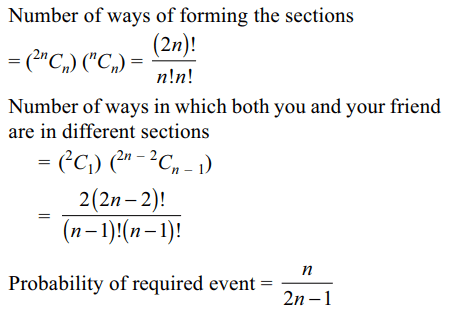4. Two persons A and B think of two numbers at random from the numbers 1, 2, …, n. Probability A think of a number smaller than thought by B is
a) $\frac{n-1}{2n}$
b) $\frac{2n-1}{2n}$
c) $\frac{n-1}{2n}$
d) $\frac{n}{2n-1}$

Explanation: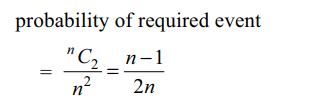5. A box contains 20 cards of these 10 have letter J printed on them and the remaining 10 have E printed on them. 3 cards are drawn from the box, the probability that we can write JEE with these cards is
a) 9/80
b) 1/8
c) 4/27
d) 15/38

Explanation: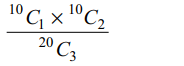6. A and B toss a coin alternatively till one of them gets a head and wins the game. If A begins the game, the probability B wins the game is
a) 1/2
b) 1/3
c) 1/4
d) 2/3

Explanation:7. A fair coin is tossed repeatedly. If head and tail appear alternatively on first 7 tosses ; then the probability that head appears on the eighth toss is
a) 1/2
b) 1/128
c) 1/256
d) 7/256

Explanation: Events are independent

8. If P(A) > 0, then the event A is independent of itself if and only if P(A) is
a) 1/3
b) 1/2
c) 1
d) $\left(\sqrt{5}-1\right)/2$

Explanation:9. Two numbers are selected at random from 1, 2, 3,…, 100 and are multiplied, then the probability (correct to two places of decimals) that the product thus obtained is divisible by 3 is
a) 0.22
b) 0.33
c) 0.44
d) 0.55